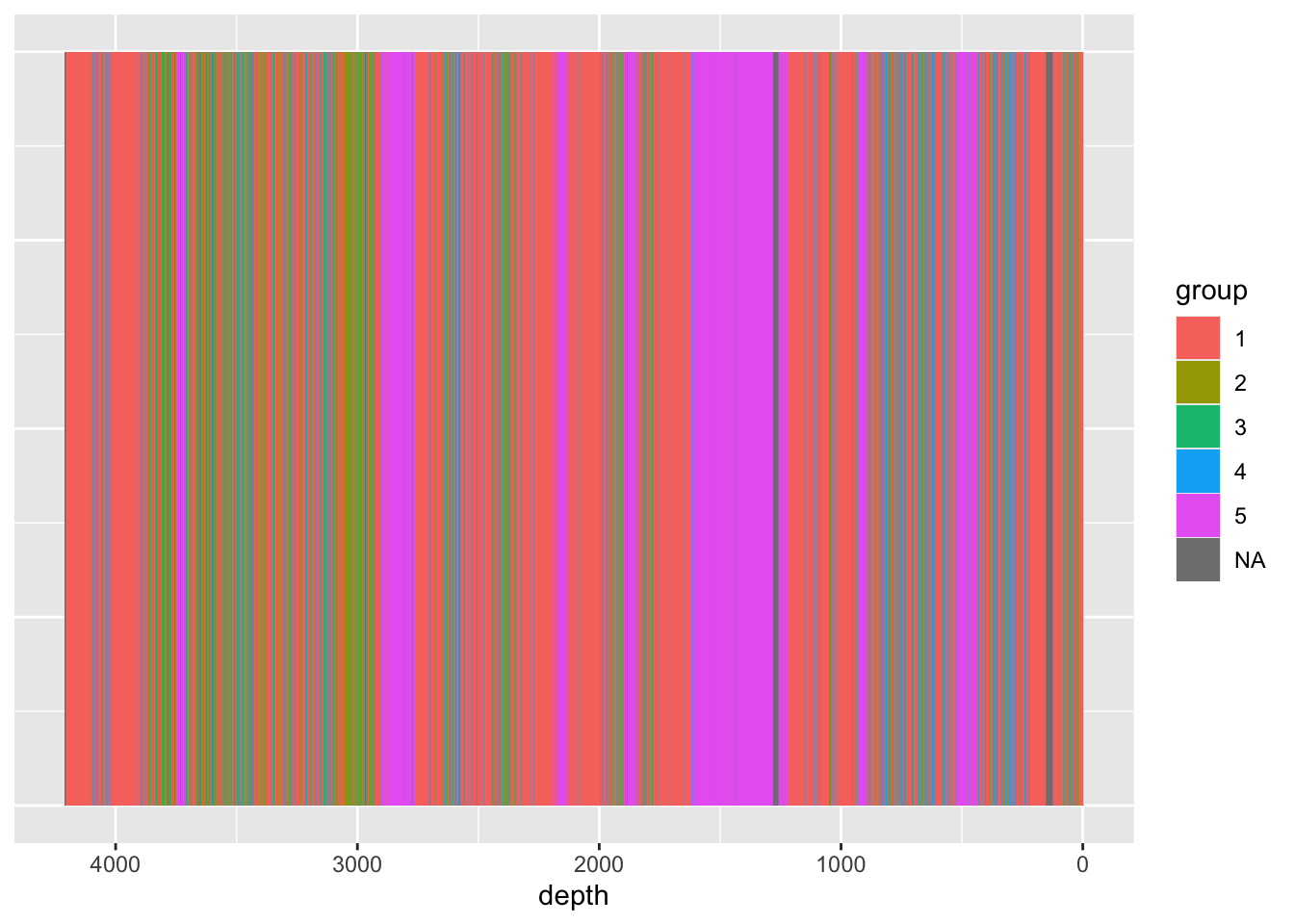## 6.4 Unconstrained Cluster Analysis

Unconstrained cluster analysis will group each measurement by similarity, returning an arbitrary number of groups as defined by the function. This is useful in identifying recurring compositional units, or identifying candidate samples for quantitative analysis.

``````left_join(CD166_19_xrf,
tibble(uid = CD166_19_xrf_acomp %>%
rownames(),
group = dist(CD166_19_xrf_acomp) %>%
hclust(method = "ward.D2") %>%
cutree(k = 5) %>%
as.factor()
),
by = "uid"
) %>%

ggplot() +
geom_tile(aes(x = depth, y = 1, fill = group)) +
scale_x_reverse() +
theme(axis.title.y = element_blank(),
axis.text.y = element_blank(),
axis.ticks.y = element_blank())``````